Second derivative test for a function of two variables

This article describes an analogue for functions of multiple variables of the following term/fact/notion for functions of one variable: second derivative test
This article describes a test that can be used to determine whether a point in the domain of a function gives a point of local, endpoint, or absolute (global) maximum or minimum of the function, and/or to narrow down the possibilities for points where such maxima or minima occur.
View a complete list of such tests

Statement

Suppose$f$ is a function of two variables$x,y$. Suppose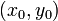$(x_0,y_0)$ is a point in the domain of$f$ such that both the first-order partial derivatives at the point are zero, i.e.,$f_x(x_0,y_0) = f_y(x_0,y_0) = 0$.

Suppose that all the second-order partial derivatives (pure and mixed) for$f$ exist and are continuous at and around$(x_0,y_0)$. Note that by Clairaut's theorem on equality of mixed partials, this implies that$f_{xy}(x_0,y_0) = f_{yx}(x_0,y_0)$.

The second derivative test helps us determine whether$f$ has a local maximum at$(x_0,y_0)$, a local minimum at$(x_0,y_0)$, or a saddle point at$(x_0,y_0)$.

First, consider the Hessian determinant of$f$ at$(x_0,y_0)$, which we define as:$D = f_{xx}(x_0,y_0)f_{yy}(x_0,y_0) - (f_{xy}(x_0,y_0))^2$

Note that this is the determinant of the Hessian matrix: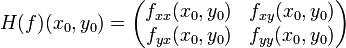$H(f)(x_0,y_0) = \begin{pmatrix} f_{xx}(x_0,y_0) & f_{xy}(x_0,y_0) \\ f_{yx}(x_0,y_0) & f_{yy}(x_0,y_0) \\\end{pmatrix}$

We now have the following:

Case Local maximum, local minimum, saddle point, or none of these? Interpretation in terms of second derivative test for a function of multiple variables$D < 0$ Saddle point The Hessian matrix is neither positive semidefinite nor negative semidefinite.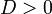$D > 0$ and$f_{xx}(x_0,y_0) > 0$ (note that these together also force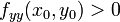$f_{yy}(x_0,y_0) > 0$) Local minimum (reasoning similar to the single-variable second derivative test) The Hessian matrix is positive definite.$D > 0$ and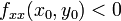$f_{xx}(x_0,y_0) < 0$ (note that these together also force$f_{yy}(x_0,y_0) < 0$) Local maximum (reasoning similar to the single-variable second derivative test) The Hessian matrix is negative definite.$D = 0$ and one or both of$f_{xx}(x_0,y_0)$ and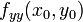$f_{yy}(x_0,y_0)$ is positive (note that if one of them is positive, the other one is either positive or zero) Inconclusive, but we can rule out the possibility of being a local maximum. The Hessian matrix is positive semidefinite but not positive definite.$D = 0$ and one or both of$f_{xx}(x_0,y_0)$ and$f_{yy}(x_0,y_0)$ is negative (note that if one of them is negative, the other one is either negative or zero) Inconclusive, but we can rule out the possibility of being a local minimum The Hessian matrix is negative semidefinite but not negative definite.
All entries of the Hessian matrix are zero, i.e.,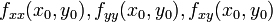$f_{xx}(x_0,y_0), f_{yy}(x_0,y_0), f_{xy}(x_0,y_0)$ are all zero Inconclusive. No possibility can be ruled out. The Hessian matrix is both positive semidefinite and negative semidefinite. Basically, we can't say anything.